# Test: 35 Year JEE Previous Year Questions: Structure of Atom

## 29 Questions MCQ Test Chemistry 35 Years JEE Main & Advanced Past year Papers | Test: 35 Year JEE Previous Year Questions: Structure of Atom

Description
Attempt Test: 35 Year JEE Previous Year Questions: Structure of Atom | 29 questions in 60 minutes | Mock test for JEE preparation | Free important questions MCQ to study Chemistry 35 Years JEE Main & Advanced Past year Papers for JEE Exam | Download free PDF with solutions
QUESTION: 1

### In a hydrogen atom, if energy of an electron in ground state is 13.6. ev, then that in the 2nd excited state is 

Solution:

2nd excited state will be the 3rd energy level.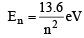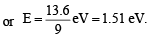QUESTION: 2

### Uncertainty in position of a minute particle of mass 25 g in space is 10–5 m. What is the uncertainty in its velocity (in ms–1) ? (h = 6.6 x 10-34 Js) 

Solution:

TIPS/Formulae :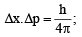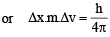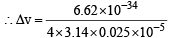= 2.1 x 10-28 ms-1

QUESTION: 3

### The number of d-electrons retained in Fe2+  (At. no. of Fe = 26) ion is

Solution:

Fe++ (26 – 2 = 24) = 1s2 2s2 2p6 3s2 3p6 4s0 3d6 hence no. of d electrons retained is 6. [Two 4s electron are removed]

QUESTION: 4

The orbital angular momentum for an electron revolving in an orbit is given by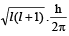This momentum for an s-electron will be given by 

Solution:

TIPS/Formulae : For s-electron, ℓ = 0

∴Orbital angular momentum =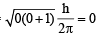QUESTION: 5

Which one of the following groupings represents a collection of isoelectronic species ?(At. nos. : Cs : 55, Br : 35)

Solution:

N3–, F and Na+ contain 10 electrons each.

QUESTION: 6

In Bohr series of lines of hydrogen spectrum, the third line from the red end corresponds to which one of the following inter-orbit jumps of the electron for Bohr orbits in an atom of hydrogen 

Planck’s constant, h = 6.63 × 10–34 Js

Solution:

The lines falling in the visible region comprise Balmer series. Hence the third line from red would be n1 =2,
n2 = 5 i.e. 5 → 2.

QUESTION: 7

The de Broglie wavelength of a tennis ball of mass 60 g moving with a velocity of 10 metres per second is approximately 

Solution: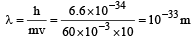QUESTION: 8

Which of the following sets of quantum numbers is correct for an electron in 4f orbital ? 

Solution:

The possible quan tum numbers for 4f electron are n = 4, ℓ = 3, m = – 3, –2 –1, 0, 1 , 2 , 3 and s =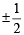Of various possiblities only option (a) is possible.

QUESTION: 9

Consider the ground state of Cr atom (X = 24). The number of electrons with the azimuthal quantum numbers, ℓ = 1 and 2 are, respectively 

Solution:

Electronic configuration of Cr atom (z = 24) = 1s2, 2s2 2p6, 3s2 3p6 3d5, 4s1
when ℓ = 1, p - subshell,
Numbers of electrons = 12
when ℓ = 2, d - subshell,
Numbers of electrons = 5

QUESTION: 10

The wavelength of the radiation emitted, when in a hydrogen atom electron falls from infinity to stationary state 1, would be (Rydberg constant = 1.097×107 m–1) 

Solution:

TIPS/Formulae :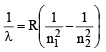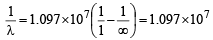λ = 91.15 x 10 -9 m ≈ 91nm

QUESTION: 11

Which one of the followin g sets of ion s represents the collection of isoelectronic species? 

(Atomic nos. : F = 9, Cl = 17, Na = 11, Mg = 12, Al = 13, K = 19, Ca = 20, Sc = 21)

Solution: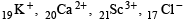each contains 18 electrons.

QUESTION: 12

In a multi-electron atom, which of the following orbitals described by the three quantum members will have the same energy in the absence of magnetic and electric fields? 
(A) n = 1, l = 0, m = 0
(B) n = 2, l = 0, m = 0
(C) n = 2, l = 1, m = 1
(D) n = 3, l = 2, m = 1
(E) n = 3, l = 2, m = 0

Solution:

The energy of an orbital is given by (n + l) in (d) and (c). (n + l) value is (3 + 2) = 5 hence they will have same energy, since there n values are also same.

QUESTION: 13

Of the following sets which one does NOT contain isoelectronic species? 

Solution:

Calculating number of electrons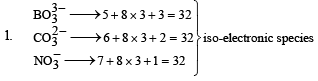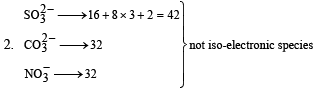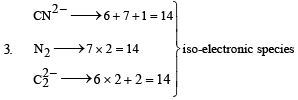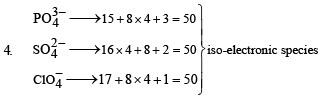Hence the species in option (b) are not isoelectronic.

QUESTION: 14

According to Bohr's theory, the angular momentum of an electron in 5th orbit is 

Solution:

Angular momentum of an electron in nth orbital is given by ,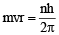For n = 5, we have

Angular momentum of electron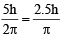QUESTION: 15

Uncertainty in the position of an electron (mass = 9.1 × 10–31 kg) moving with a velocity 300 ms–1, accurate upto 0.001% will be 

(h = 6.63 × 10–34 Js)

Solution:

Given m = 9.1 × 10–31kg , h = 6.6 × 10–34Js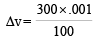= 0.003ms–1

From Heisenberg's uncertainity principle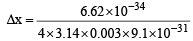= 1.92 x 10-2 m

QUESTION: 16

Which one of the following sets of ions represents a collection of isoelectronic species? 

Solution:

(a)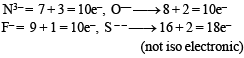(b)  Li+ = 3+1= 4e,  Na+ = 11–1 = 10e,
Mg++  = 12–2=10e
Ca++ = 20 – 2 = 18e–       (not isoelectronic)

(c) K+ = 19 – 1= 18e, Cl =17 + 1  = 18e,
Ca++ = 20 – 2 =18e, Sc3+ = 21–3 = 18e
(isoelectronic)

(d) Ba++ 56 – 2 = 54e,  Sr++ 38–2 = 36e–
K+= 9–1 = 18e,  Ca++= 20–2 = 18e
(not  isoelectronic)

QUESTION: 17

Which of the following sets of quantum numbers represents the highest energy of an atom? 

Solution:

(a) n = 3, ℓ = 0 means 3s-orbital and n + ℓ = 3
(b) n = 3, ℓ = 1 means 3p-orbital n + ℓ = 4
(c) n = 3, ℓ = 2 means 3d-orbital n + ℓ = 5
(d) n = 4, ℓ = 0 means 4s-orbital n + ℓ = 4
Increasing order of energy among these orbitals is 3s < 3p < 4s < 3d
∴ 3d has highest energy..

QUESTION: 18

Which one of the following constitutes a group of the isoelectronic species? 

Solution:

Species having same n umber of el ectr on s ar e isoelectronic calculating the number of electrons in each species given here, we get.
CN (6 + 7 + 1 = 14); N2 (7 + 7 = 14);
O22–(8 + 8 +2 = 18) ; C22– (6 + 6 + 2 = 14);
O2 (8 + 8 + 1 = 17) ; NO+ (7 + 8 – 1 = 14)
CO (6 + 8 = 14) ; NO (7 + 8 = 15)
From the above calculation we find that all the species listed in choice (b) have 14 electrons each so it is the correct answer.

QUESTION: 19

The ionization enthalpy of hydrogen atom is 1.312 × 106 J mol–1. The energy required to excite the electron in the atom from n = 1 to n = 2 is 

Solution:

(ΔE), The energy required to excite an electron in an atom of hydrogen from n = 1 to n = 2 is ΔE  (difference in energy E2 and E1) Values of E2 and E1 are, E1 = – 1.312 × 106 J mol–1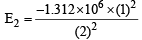= –3.28 × 105 J mol–1

ΔE is given by the relation,

∴ ΔE = E2 – E1  = [–3.28 × 105]– [–1.312 × 106 ] J mol–1

= (–3.28 × 105 + 1.312 × 106) J mol–1

= 9.84 × 105 J mol–1

Thus the correct answer is (d)

QUESTION: 20

Calculate the wavelength (in nanometer) associated with a proton moving at 1.0 × 103 ms –1. (Mass of proton = 1.67 × 10–27 kg and  h = 6.63 × 10–34 Js)

Solution: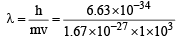= 3.97 × 10–10 meter  = 0.397 nanometer

QUESTION: 21

In an atom, an electron is moving with a speed of 600 m/s with an accuracy of 0.005%. Certainity with which the position of the electron can be located is ( h = 6.6 × 10–34 kg m2s–1, mass of electron, em = 9.1 × 10–31 kg) : 

Solution:

According to Heisenberg uncertainty principle.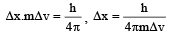Here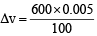= 0.03

So,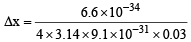= 1.92 × 10–3 meter

QUESTION: 22

The energy required to break one mole of Cl – Cl bonds in Cl2 is 242 kJ mol–1. The longest wavelength of light capable of breaking a single Cl – Cl bond is (c = 3 × 108 ms–1 and NA = 6.02 × 1023 mol–1). 

Solution:

Energy required to break one mole of Cl – Cl bonds in Cl2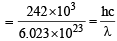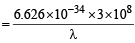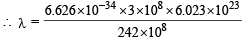= 0.4947 × 10–6 m  = 494.7 nm

QUESTION: 23

Ionisation energy of He+ is 19.6 × 10–18 J atom–1. The energy of the first stationary state (n = 1) of Li2+ is 

Solution: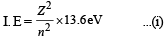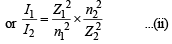Given I1 = – 19.6 × 10–18 , Z1 = 2, n1 = 1 , Z2 = 3 and n2 = 1

Substituting these values in equation (ii).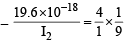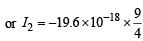= – 4.41 × 10–17 J/atom

QUESTION: 24

A gas a bsor bs a ph oton of 355 n m an d emits at two wavelengths. If one of the emissions is at 680 nm, the other is at:

Solution:

Energy of absorbed photon = Sum of the energies of emitted photon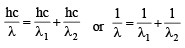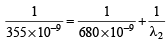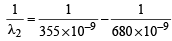= 1.346 x106

or λ2= 1/1.346 × 106 = 743 × 10–9m = 743 nm

QUESTION: 25

The electrons identified by quantum numbers n and l :
(A) n = 4, l = 1
(B) n = 4, l = 0
(C) n = 3, l = 2
(D) n = 3, l = 1
can be placed in order of increasing energy as : 

Solution:

(a) 4 p   (b)  4 s (c) 3 d (d)   3 p

Accroding to Bohr Bury's (n + l) rule, increasing order of energy (D) < (B) < (C) < (A).
Note : If the two orbitals have same value of (n + l) then the orbital with lower value of n will be filled first.

QUESTION: 26

Energy of an electron is given by E = – 2.178 ×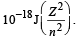Wavelength of light required to excite an electron in an hydrogen atom from level n = 1 to n = 2 will be : (h = 6.62 × 10 –34 Js and c = 3.0 × 108 ms–1) [JEE M 2013]

Solution: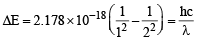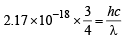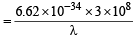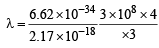= 1.214 × 10–7m

QUESTION: 27

The correct set of four quantum numbers for the valence electrons of rubidium atom (Z = 37) is: [JEE M 2014]

Solution:

The electronic configuration of Rubidium (Rb = 37) is

1s22s2 2 p6 3s23 p6 3d10 4s2 4 p6 5s1
Since last electron enters in 5s orbital Hence n = 5, l = 0, m = 0, s =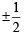QUESTION: 28

Which of the following is the energy of a possible excited state of hydrogen ? [JEE M 2015]

Solution:

Total energy =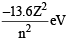where n = 2, 3, 4 ....
Putting n = 2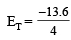= -3.4eV

QUESTION: 29

A stream of electrons from a heated filaments was passed two charged plates kept at a potential difference V esu. If e and m are charge and mass of an electron, respectively, then the value of h/λ (where λ is wavelength associated with electron wave) is given by: [JEE M 2016]

Solution:

As electron of charge 'e' is passed through 'V' volt, kinetic energy of electron will be eV

Wavelength of electron wave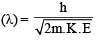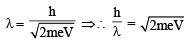Use Code STAYHOME200 and get INR 200 additional OFF Use Coupon Code